# Demystifying Shapeless

Aug. 16, 2014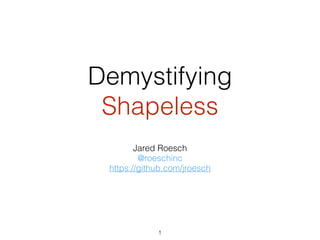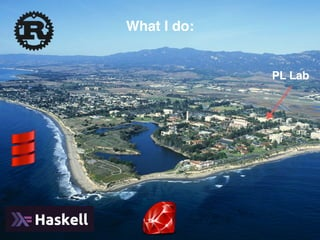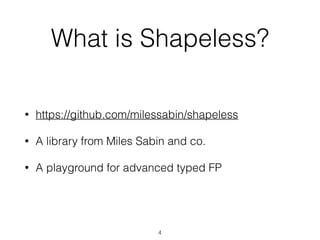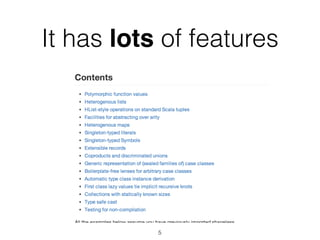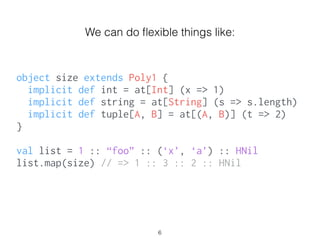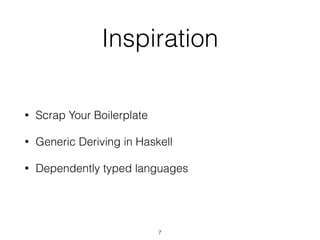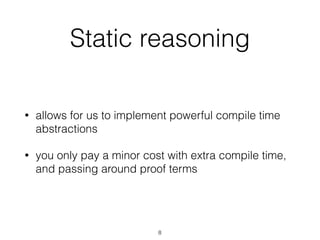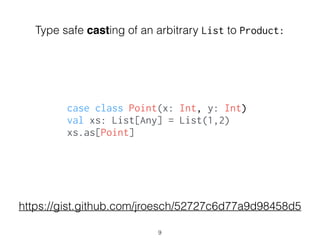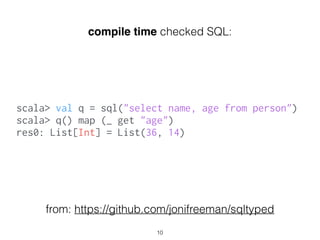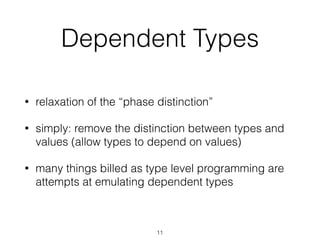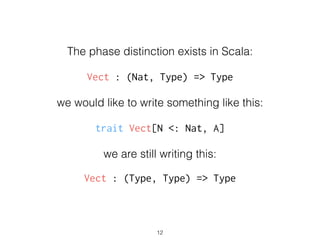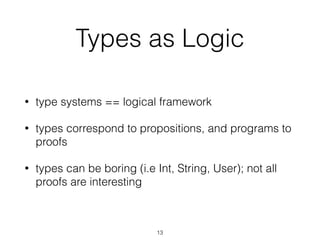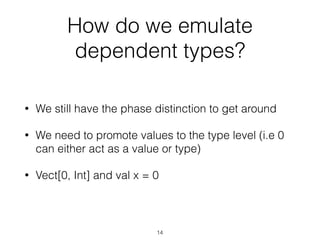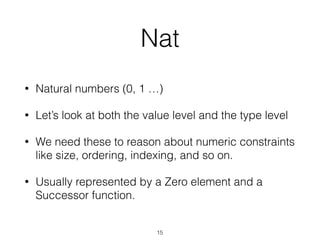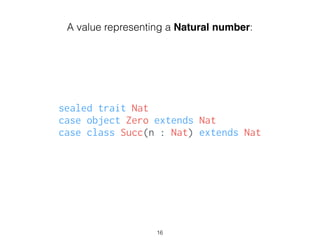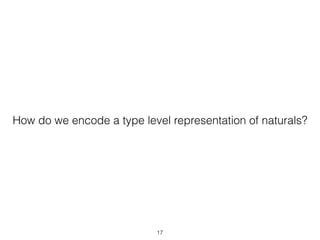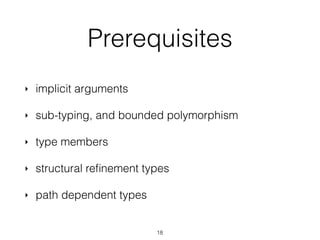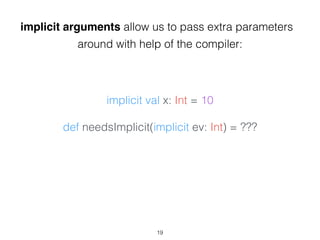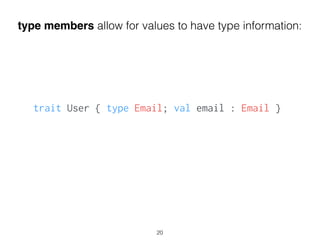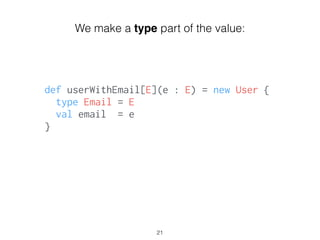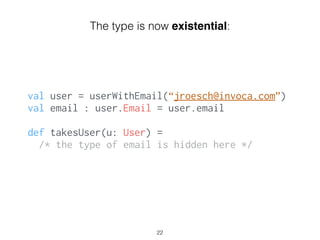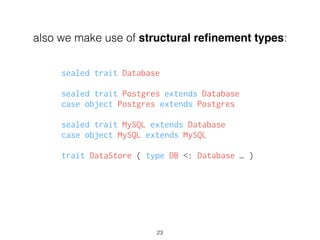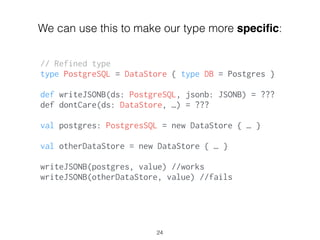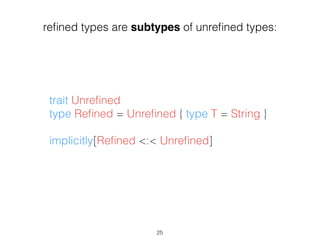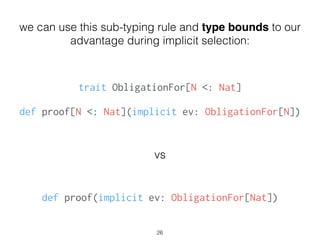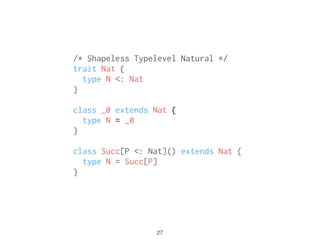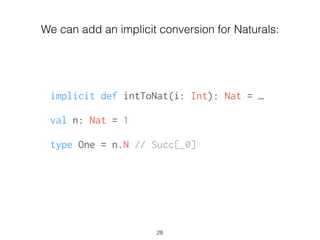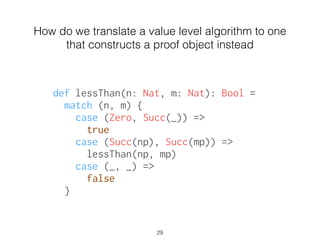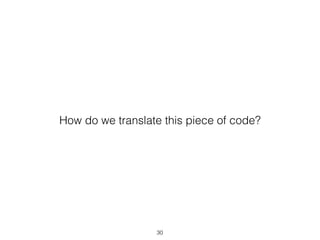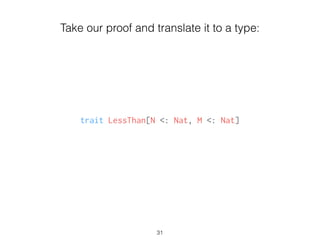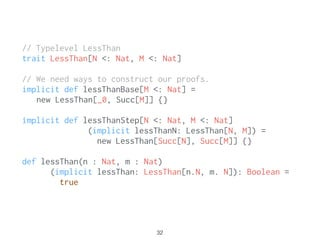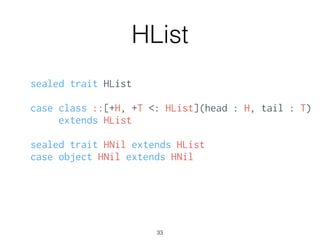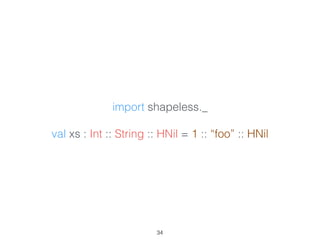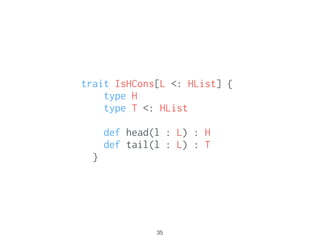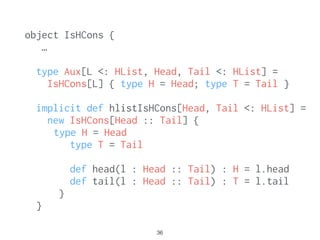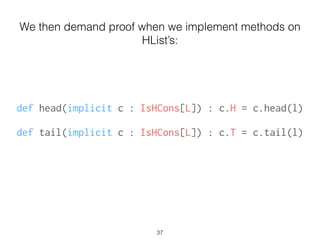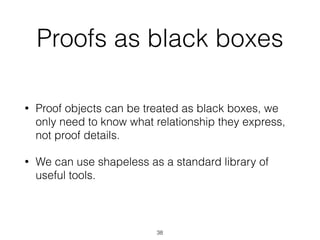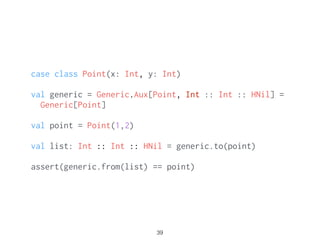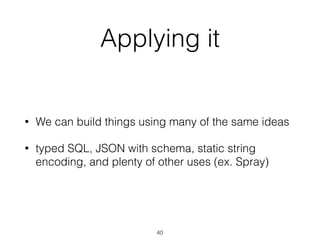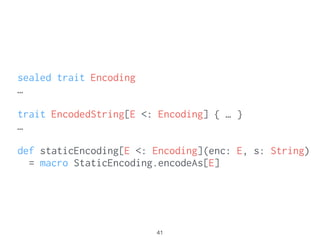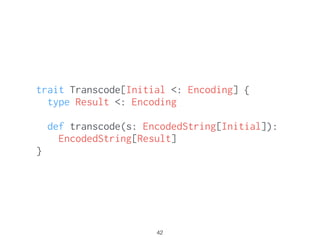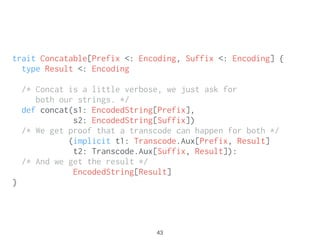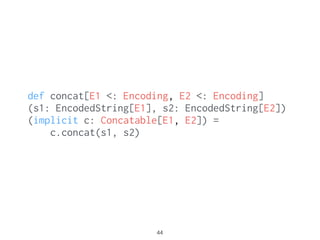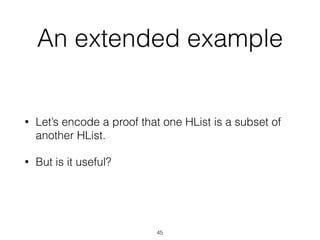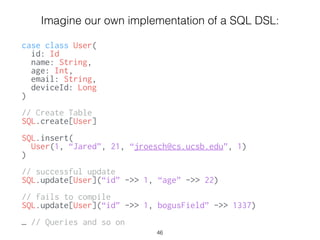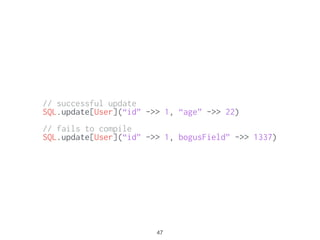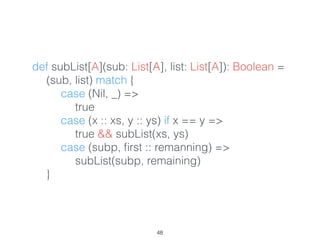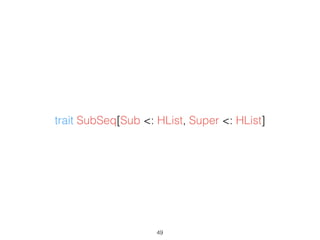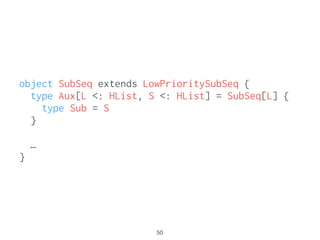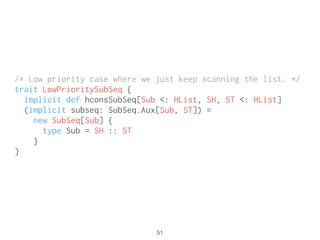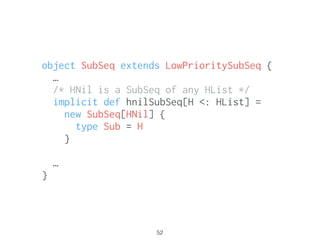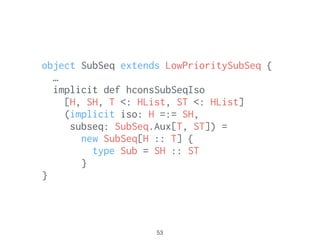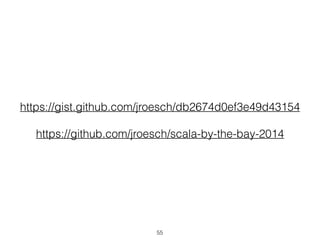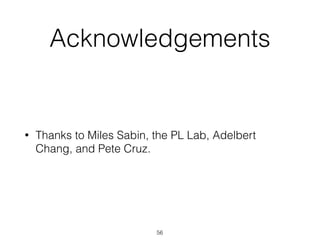1 of 57

### Demystifying Shapeless

1. Demystifying Shapeless Jared Roesch @roeschinc https://github.com/jroesch 1
2. Who I am 2
3. What I do: PL Lab 3
4. What is Shapeless? • https://github.com/milessabin/shapeless • A library from Miles Sabin and co. • A playground for advanced typed FP 4
5. It has lots of features 5
6. object size extends Poly1 { implicit def int = at[Int] (x => 1) implicit def string = at[String] (s => s.length) implicit def tuple[A, B] = at[(A, B)] (t => 2) } ! val list = 1 :: “foo” :: (‘x’, ‘a’) :: HNil list.map(size) // => 1 :: 3 :: 2 :: HNil We can do ﬂexible things like: 6
7. Inspiration • Scrap Your Boilerplate • Generic Deriving in Haskell • Dependently typed languages 7
8. Static reasoning • allows for us to implement powerful compile time abstractions • you only pay a minor cost with extra compile time, and passing around proof terms 8
9. Type safe casting of an arbitrary List to Product: 9 case class Point(x: Int, y: Int) val xs: List[Any] = List(1,2) xs.as[Point] https://gist.github.com/jroesch/52727c6d77a9d98458d5
10. scala> val q = sql("select name, age from person") scala> q() map (_ get "age") res0: List[Int] = List(36, 14) compile time checked SQL: from: https://github.com/jonifreeman/sqltyped 10
11. Dependent Types • relaxation of the “phase distinction” • simply: remove the distinction between types and values (allow types to depend on values) • many things billed as type level programming are attempts at emulating dependent types 11
12. The phase distinction exists in Scala: ! Vect : (Nat, Type) => Type ! we would like to write something like this: ! trait Vect[N <: Nat, A] ! we are still writing this: ! Vect : (Type, Type) => Type 12
13. Types as Logic • type systems == logical framework • types correspond to propositions, and programs to proofs • types can be boring (i.e Int, String, User); not all proofs are interesting 13
14. How do we emulate dependent types? • We still have the phase distinction to get around • We need to promote values to the type level (i.e 0 can either act as a value or type) • Vect[0, Int] and val x = 0 14
15. Nat • Natural numbers (0, 1 …) • Let’s look at both the value level and the type level • We need these to reason about numeric constraints like size, ordering, indexing, and so on. • Usually represented by a Zero element and a Successor function. 15
16. sealed trait Nat case object Zero extends Nat case class Succ(n : Nat) extends Nat 16 A value representing a Natural number:
17. How do we encode a type level representation of naturals? 17
18. Prerequisites ‣ implicit arguments ‣ sub-typing, and bounded polymorphism ‣ type members ‣ structural reﬁnement types ‣ path dependent types 18
19. implicit val x: Int = 10 ! def needsImplicit(implicit ev: Int) = ??? implicit arguments allow us to pass extra parameters around with help of the compiler: 19
20. type members allow for values to have type information: trait User { type Email; val email : Email } ! 20
21. def userWithEmail[E](e : E) = new User { type Email = E val email = e } ! 21 We make a type part of the value:
22. val user = userWithEmail(“jroesch@invoca.com”) val email : user.Email = user.email ! def takesUser(u: User) = /* the type of email is hidden here */ 22 The type is now existential:
23. also we make use of structural reﬁnement types: sealed trait Database ! sealed trait Postgres extends Database case object Postgres extends Postgres ! sealed trait MySQL extends Database case object MySQL extends MySQL ! trait DataStore { type DB <: Database … } 23
24. // Refined type type PostgreSQL = DataStore { type DB = Postgres } ! def writeJSONB(ds: PostgreSQL, jsonb: JSONB) = ??? def dontCare(ds: DataStore, …) = ??? ! val postgres: PostgresSQL = new DataStore { … } ! val otherDataStore = new DataStore { … } ! writeJSONB(postgres, value) //works writeJSONB(otherDataStore, value) //fails 24 We can use this to make our type more speciﬁc:
25. trait Unreﬁned type Reﬁned = Unreﬁned { type T = String } ! implicitly[Reﬁned <:< Unreﬁned] 25 reﬁned types are subtypes of unreﬁned types:
26. trait ObligationFor[N <: Nat] ! def proof[N <: Nat](implicit ev: ObligationFor[N]) 26 we can use this sub-typing rule and type bounds to our advantage during implicit selection: vs def proof(implicit ev: ObligationFor[Nat])
27. /* Shapeless Typelevel Natural */ trait Nat { type N <: Nat } ! class _0 extends Nat { type N = _0 } ! class Succ[P <: Nat]() extends Nat { type N = Succ[P] } 27
28. implicit def intToNat(i: Int): Nat = … ! val n: Nat = 1 ! type One = n.N // Succ[_0] 28 We can add an implicit conversion for Naturals:
29. def lessThan(n: Nat, m: Nat): Bool = match (n, m) { case (Zero, Succ(_)) => true case (Succ(np), Succ(mp)) => lessThan(np, mp) case (_, _) => false } How do we translate a value level algorithm to one that constructs a proof object instead 29
30. How do we translate this piece of code? 30
31. trait LessThan[N <: Nat, M <: Nat] 31 Take our proof and translate it to a type:
32. // Typelevel LessThan trait LessThan[N <: Nat, M <: Nat] ! // We need ways to construct our proofs. implicit def lessThanBase[M <: Nat] = new LessThan[_0, Succ[M]] {} ! implicit def lessThanStep[N <: Nat, M <: Nat] (implicit lessThanN: LessThan[N, M]) = new LessThan[Succ[N], Succ[M]] {} ! def lessThan(n : Nat, m : Nat) (implicit lessThan: LessThan[n.N, m. N]): Boolean = true 32
33. HList sealed trait HList ! case class ::[+H, +T <: HList](head : H, tail : T) extends HList ! sealed trait HNil extends HList case object HNil extends HNil 33
34. import shapeless._ ! val xs : Int :: String :: HNil = 1 :: “foo” :: HNil 34
35. trait IsHCons[L <: HList] { type H type T <: HList def head(l : L) : H def tail(l : L) : T } 35
36. object IsHCons { … ! type Aux[L <: HList, Head, Tail <: HList] = IsHCons[L] { type H = Head; type T = Tail } ! implicit def hlistIsHCons[Head, Tail <: HList] = new IsHCons[Head :: Tail] { type H = Head type T = Tail def head(l : Head :: Tail) : H = l.head def tail(l : Head :: Tail) : T = l.tail } } 36
37. def head(implicit c : IsHCons[L]) : c.H = c.head(l) ! def tail(implicit c : IsHCons[L]) : c.T = c.tail(l) We then demand proof when we implement methods on HList’s: 37
38. Proofs as black boxes • Proof objects can be treated as black boxes, we only need to know what relationship they express, not proof details. • We can use shapeless as a standard library of useful tools. 38
39. case class Point(x: Int, y: Int) ! val generic = Generic.Aux[Point, Int :: Int :: HNil] = Generic[Point] ! val point = Point(1,2) ! val list: Int :: Int :: HNil = generic.to(point) ! assert(generic.from(list) == point) 39
40. Applying it • We can build things using many of the same ideas • typed SQL, JSON with schema, static string encoding, and plenty of other uses (ex. Spray) 40
41. 41 sealed trait Encoding … ! trait EncodedString[E <: Encoding] { … } … ! def staticEncoding[E <: Encoding](enc: E, s: String) = macro StaticEncoding.encodeAs[E]
42. 42 trait Transcode[Initial <: Encoding] { type Result <: Encoding ! def transcode(s: EncodedString[Initial]): EncodedString[Result] }
43. 43 trait Concatable[Prefix <: Encoding, Suffix <: Encoding] { type Result <: Encoding /* Concat is a little verbose, we just ask for both our strings. */ def concat(s1: EncodedString[Prefix], s2: EncodedString[Suffix]) /* We get proof that a transcode can happen for both */ (implicit t1: Transcode.Aux[Prefix, Result] t2: Transcode.Aux[Suffix, Result]): /* And we get the result */ EncodedString[Result] }
44. 44 def concat[E1 <: Encoding, E2 <: Encoding] (s1: EncodedString[E1], s2: EncodedString[E2]) (implicit c: Concatable[E1, E2]) = c.concat(s1, s2)
45. An extended example • Let’s encode a proof that one HList is a subset of another HList. • But is it useful? 45
46. Imagine our own implementation of a SQL DSL: ! case class User( id: Id name: String, age: Int, email: String, deviceId: Long ) ! // Create Table SQL.create[User] ! SQL.insert( User(1, “Jared”, 21, “jroesch@cs.ucsb.edu”, 1) ) ! // successful update SQL.update[User](“id” ->> 1, “age” ->> 22) ! // fails to compile SQL.update[User](“id” ->> 1, bogusField” ->> 1337) ! … // Queries and so on 46
47. // successful update SQL.update[User](“id” ->> 1, “age” ->> 22) ! // fails to compile SQL.update[User](“id” ->> 1, bogusField” ->> 1337) 47
48. 48 def subList[A](sub: List[A], list: List[A]): Boolean = (sub, list) match { case (Nil, _) => true case (x :: xs, y :: ys) if x == y => true && subList(xs, ys) case (subp, ﬁrst :: remanning) => subList(subp, remaining) }
49. 49 trait SubSeq[Sub <: HList, Super <: HList]
50. 50 object SubSeq extends LowPrioritySubSeq { type Aux[L <: HList, S <: HList] = SubSeq[L] { type Sub = S } … }
51. 51 /* Low priority case where we just keep scanning the list. */ trait LowPrioritySubSeq { implicit def hconsSubSeq[Sub <: HList, SH, ST <: HList] (implicit subseq: SubSeq.Aux[Sub, ST]) = new SubSeq[Sub] { type Sub = SH :: ST } }
52. 52 object SubSeq extends LowPrioritySubSeq { … /* HNil is a SubSeq of any HList */ implicit def hnilSubSeq[H <: HList] = new SubSeq[HNil] { type Sub = H } ! … }
53. 53 object SubSeq extends LowPrioritySubSeq { … implicit def hconsSubSeqIso [H, SH, T <: HList, ST <: HList] (implicit iso: H =:= SH, subseq: SubSeq.Aux[T, ST]) = new SubSeq[H :: T] { type Sub = SH :: ST } }
54. 54 There are few ways to improve upon our last example, I’ll leave it as a fun puzzle for you.
55. 55 https://gist.github.com/jroesch/db2674d0ef3e49d43154 ! https://github.com/jroesch/scala-by-the-bay-2014
56. Acknowledgements • Thanks to Miles Sabin, the PL Lab, Adelbert Chang, and Pete Cruz. 56
57. Thank you for your time, questions? 57